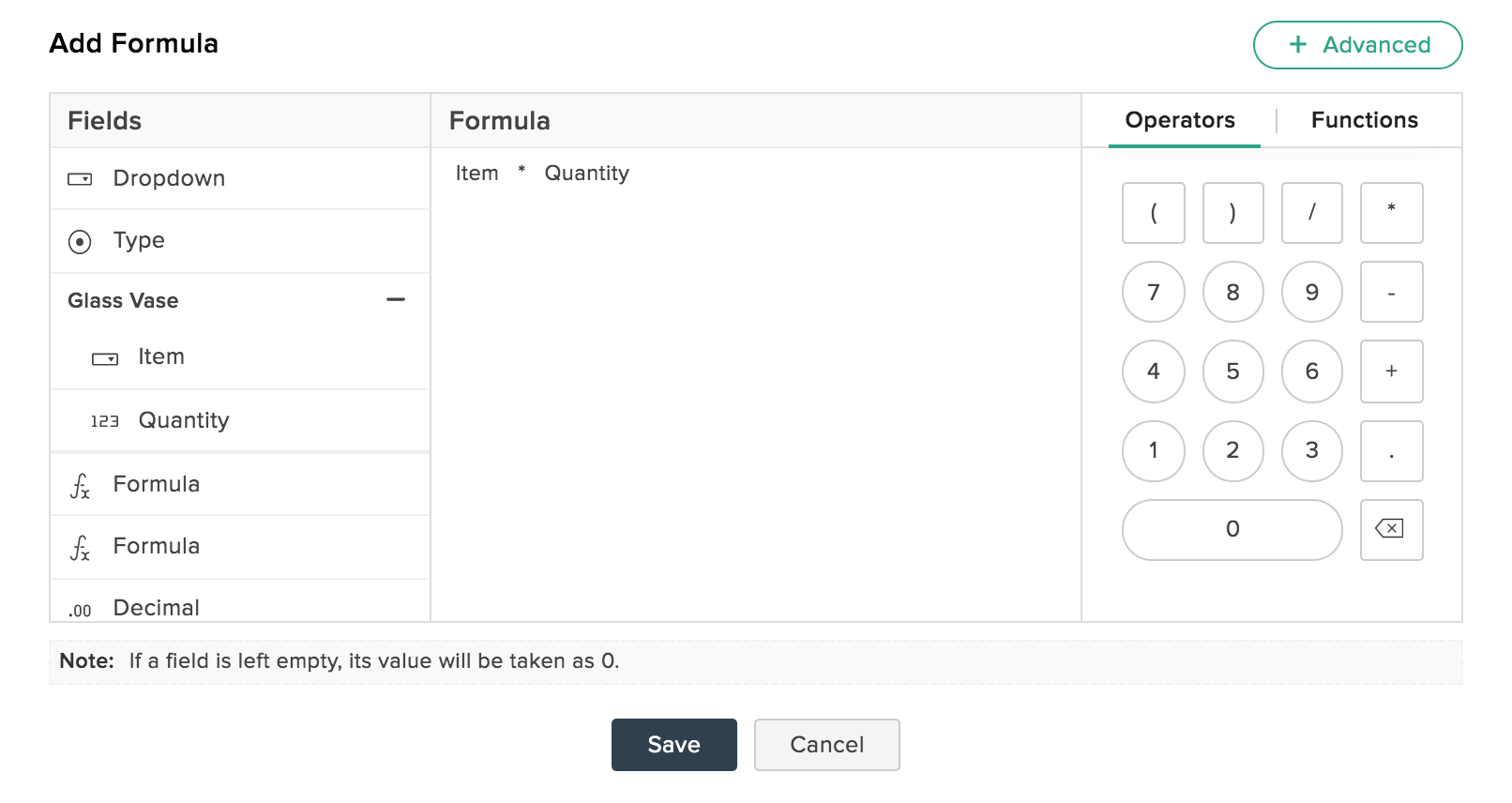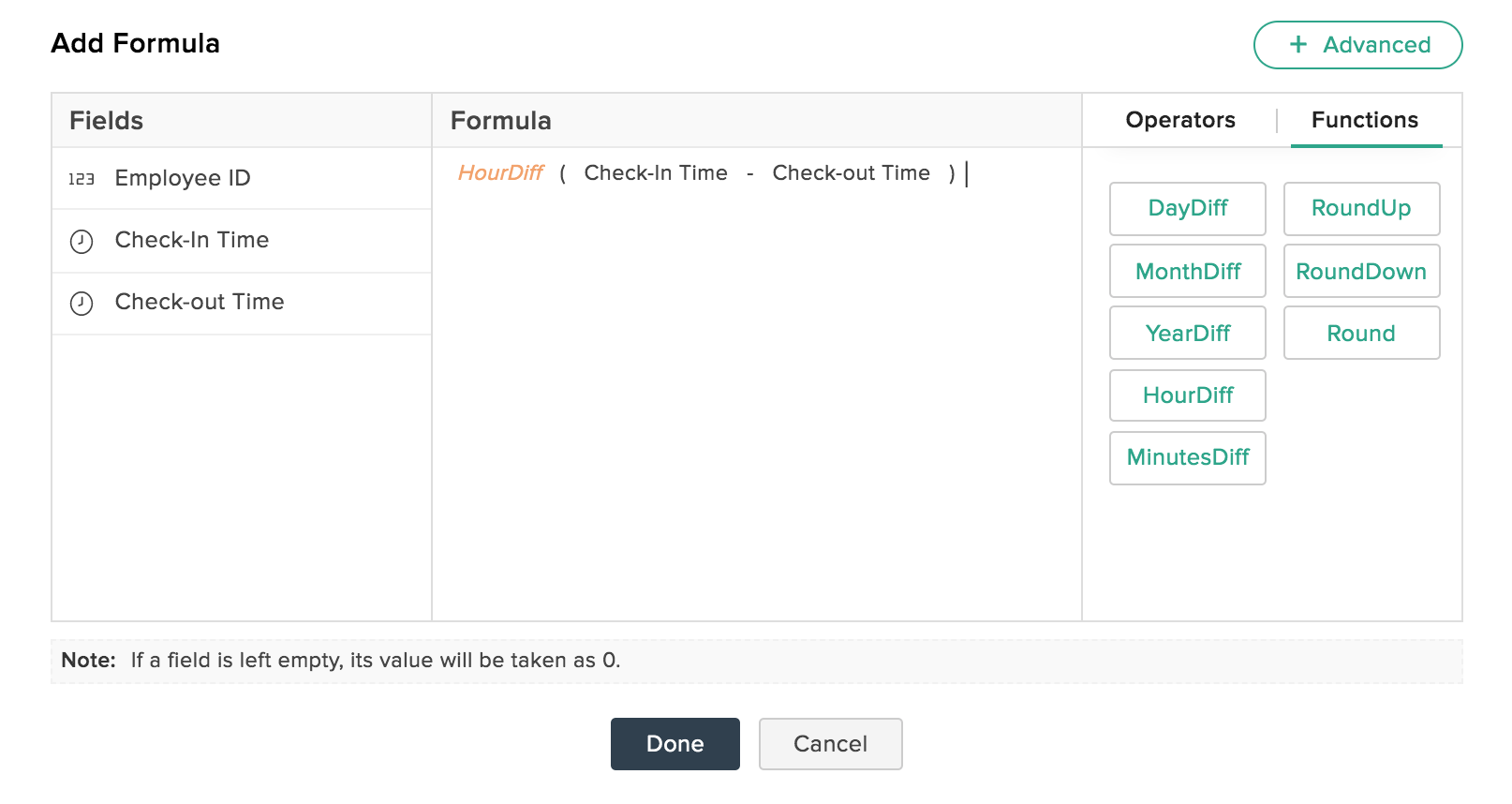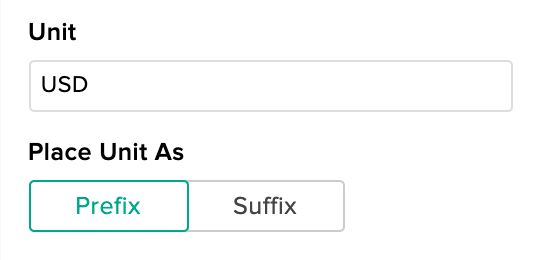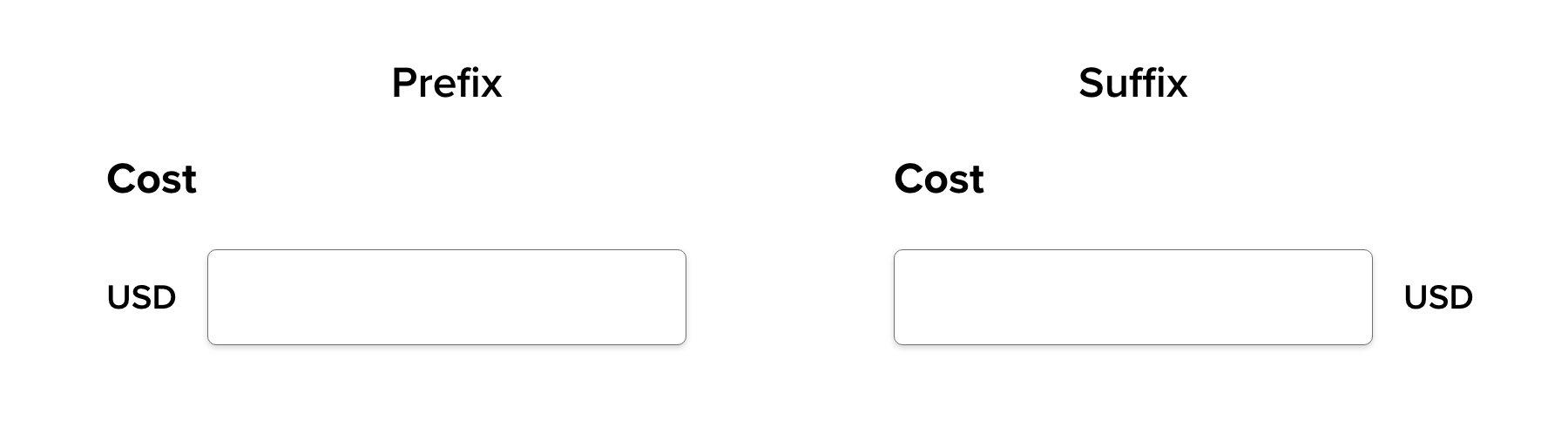# Perform Calculations

Make your forms a math wiz by allowing it to perform calculations. You can use this to total up costs, process grade quizzes, discounts, and more!

## Introduction

The Formula field is to be used to set up any form calculation. When your respondents fill in the form, calculations would be performed based on their input and the answer is displayed in the field you have entered your formula in.

Using this you can,

• Perform mathematical operations such as addition, subtraction, multiplication, and division.
• Use certain math functions to calculate differences between two times, dates, etc.
• Set up Conditional Calculations to perform calculations based on the respondent's answers.

## Setup

To include a formula in your form:

1. Click the Formula field under the Advanced Fields section.
2. In the pop-up, your form's fields would appear in the left pane. Use the fields, numbers, and operators to come up with your formula.
3. You can also choose to hide this field from your respondents by clicking on the Hide Field checkbox. You will find it under the Properties pane in the builder.
4. If you wish to edit your formula, click on the Edit link under the Properties pane.Along with the mathematical Operators, you can use the form fields inside the calculation. The field types that we support for the Formula field are Number, Decimal, Currency, Slider, Rating, Formula, Radio, Checkbox, Multiple Choice, Dropdown, Image Choice, Date, Date-Time, Time.

For example, you can calculate the amount your customer has to pay using the formula field and display the amount in the payment field automatically. For this, you can set the formula as Item * Quantity, where the number value assigned for the field Item is taken for the calculation.

Note: While using the Formula field as a variable for calculating another formula, ensure the two formulas aren't mutually dependent. If you've come across the error because two formulas being mutually dependent, then this is the problem is as outlined below. For example, n1, n2, n3 are three number fields, and f1, f2, f3 are the formulas that you've created where,

f1= n1+ n2
f2= n3 + f3
f3= f1 + f2

Formula 2 depends on Formula 3's answer (and vice versa) to perform calculations, resulting in an endless loop. Recheck your formula to ensure you avoid this type of infinite loop.

## Functions

Using the math functions we support, you can calculate the differences between time and date fields, and also round off decimal numbers into integers. You will find these options under Functions in the right pane of the Formula field pop-up.

To use a function:

• Tap on the math function you want to add.
• It generates a syntax like this:  Function (Field Name - Field Name).
• Replace the field's placeholders with the required form fields by clicking them from the left pane.The math function we support are:

 Function Role Supported Field Types DayDiff Returns the day difference between two date values Date, Date-Time MonthDiff Returns the month difference between two date values Date, Date-Time YearDiff Returns the year difference between two date values Date, Date-Time HourDiff Returns the hour difference between two time values Time, Date-Time MinutesDiff Returns the minute difference between two time values Time, Date-Time RoundUp Rounds off the decimal number to that next value, away from zero Decimal, Currency, Formula RoundDown Rounds off the decimal number to that of the previous value Decimal, Currency, Formula Round Rounds off a decimal number to that of the next number when the decimal value is .5 or above, and to the previous number when the decimal value is below .5 Decimal, Currency, Formula

## ​Adding a Unit to Formula

You can add a unit to your formula to show the result more contextually in the live form. A unit can be added once the formula configuration is completed.

To include a unit for the formula calculated value:

1. Enter a suitable Unit under Properties.1. You can select to position this unit either as a Prefix or a SuffixNote : The unit for a formula will be displayed only in Subform's Popup mode.